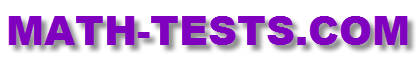#Are you looking for an 8th grade math test that you can take online? The following online quizzes and tests are based on the eighth grade math standards.

These online tests are designed to work on computers, laptops, iPads, and other tablets. There is no need to download any app for these activities.

Exponents Math Test
Identify and use exponents in an expression.

Negative Exponents Math Test
Simply an expression involving negative exponents.

Multiply and Divide with Exponents Math Test
Solve expressions involving exponents by multiplying and dividing.

Angles Math Test
Identify types of angles and solve equations based upon characteristics of transverse lines and parallel lines.

Slope Math Test
Calculate the slope of a line through two points. Calculate the slope based on a line drawn on a graph.

Return from the 8th Grade Math Test Page to the Math Tests homepage.